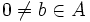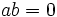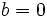# Reduced Noetherian implies zero divisor in minimal prime

This article defines a result where the base ring (or one or more of the rings involved) is Noetherian
View more results involving Noetherianness or Read a survey article on applying Noetherianness

## Statement

Suppose$A$ is a reduced Noetherian ring: a reduced ring that is also a Noetherian ring: in other words, it has no nilpotents, and every ideal is finitely generated. Then, any zero divisor in$A$ must be inside some minimal prime ideal of$A$.

(For non-Noetherian rings, we can only say that every zero divisor must be inside some prime ideal, since minimal primes are not guaranteed to exist).

## Related facts

### Converse

The converse is true for any Noetherian ring. Further information: Noetherian implies every element in minimal prime is zero divisor

## Facts used

1. Noetherian ring has finitely many minimal primes and every prime contains a minimal prime (and thus, in particular, the nilradical is an intersection of those finitely many minimal primes)
2. Equivalence of definitions of nilradical: In a commutative unital ring, the nilradical, defined as the set of nilpotent elements, also equals the intersection of all prime ideals.

## Generalizations

The result is true if we replace the assumption of Noetherianness by the assumption that every prime contains a minimal prime.

## Proof

Given: Suppose$a \in A$ is a zero divisor

To prove:$a$ is in some minimal prime.

Proof: There exists$0 \ne b \in A$ such that$ab = 0$.

1. By fact (1), every prime ideal contains a minimal prime ideal. Hence, the intersection of all prime ideals equals the intersection of all minimal prime ideals. By fact (2), the intersection of all prime ideals is the set of nilpotent elements. Finally, since the ring is reduced, it has no nonzero nilpotents, so the intersection of all primes is the zero ideal. Thus, the intersection of all minimal primes is the zero ideal.
2. Clearly, we have that$ab$ is in every minimal prime. For every minimal prime, either$a$ is in the minimal prime or$b$ is in the minimal prime. If$a$ is not in any minimal prime, then$b$ must be in every minimal prime, hence$b$ must be in the intersection of all minimal primes.
3. Combining steps (1) and (2), we obtain that$b = 0$.